# The Universe of Discourse

Fri, 14 Aug 2015

Earlier articles: Introduction Common features Page 1 (numerals) Page 2 (arithmetic)

This is page 3 of the Cosmic Call message. An explanation follows.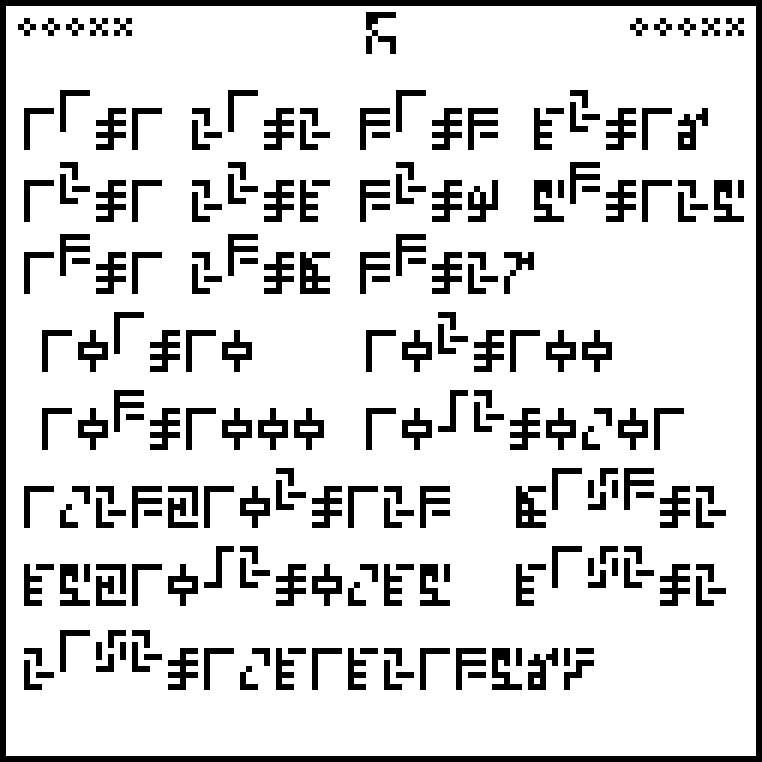Reminder: page 1 explained the ten digits: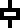0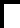1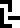2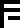3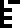4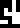5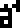6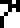7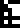8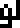9

And the equal sign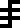. Page 2 explained the four basic arithmetic operations and some associated notions: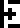addition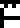subtraction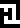multiplication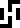division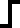negation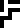ellipsis (…)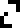decimalpoint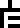indeterminate

This page, headed with the glyph for “mathematics”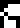, explains notations for exponentiation and scientific notation. (This notation was first used on page 1 in the mersenne prime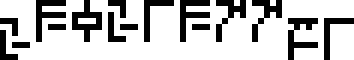.)

Exponentiation could be represented by an operator, but instead the authors have chosen to represent it by a superscripted position on the page, as is done in conventional mathematical notation. This saves space.

The top section of the page has small examples of exponentiation, including for example !!5^3=125!!: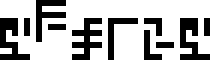!!5^3=125!!

There is a section that follows with powers of 10: !!10^1=10, 10^2=100, 10^3=1000, !! and more interestingly !!10^{{}^-2} = 0.01!!: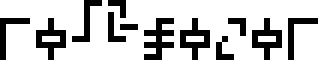!!10^{{}^-2} = 0.01!!

This is a lead-in to the next section, which expresses various quantities in scientific notation, which will recur frequently later on. For example, !!0.045!! can be written as !!45\times 10^{{}^-2}!!: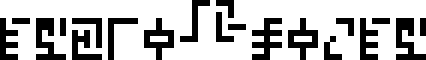!!45\times10^{{}^-2} = 0.45!!

Finally, there is an offhand remark about the approximate value of the square root of 2: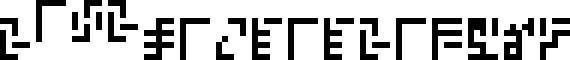!!2^{1\div 2} = 1.41421356…!!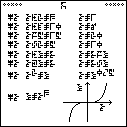The next article will discuss page 4, shown at right. (Click to enlarge.) Try to figure it out before then.